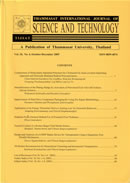THAMMASAT INTERNATIONAL JOURNAL OF SCIENCE & TECHNOLOGY

Volume 25, No. 01, Month JANUARY, Year 2020, Pages 95 - 105

An approach to identify significant parameters in blood flow through human arteries

Karthik Alasakani, Sai Lakshmi Radhika Tantravahi, Praveen Kumar

Abstract

In this work, we attempt to identify parameters that are significant in blood flow through human arteries. For this, we developed a mathematical model and carried out simulations using data on human arteries and then performed a statistical analysis of the data generated. The mathematical model considers blood as a Newtonian fluid with its constitutive equation comprising two parameters termed as fluid parameters, namely the density and viscosity. Arteries are modelled as tapered and elastic circular pipes wherein the radius of the artery is in terms of the material parameters, termed taper fraction, expanding/contracting parameter and aspect ratio (=radius of the artery/length of the artery). Blood flow is described as pulsatile with the frequency of oscillations as the flow parameter monitored. The resulting system of partial differential equations is solved using the Homotopy Analysis Method (HAM). Data on human arteries (healthy) has been taken from literature, and we worked on different age groups ranging from 15 years to 65 years, both on male and female populations. The two physical quantities of interest i.e. the wall shear stress (WSS) and the volumetric flow rate have been computed and analysis has been carried out to identify significant parameters using a multiple linear regression model.

Keywords

Blood flow, HAM, Human artery, Mathematical model, Newtonian fluid, Statistical analysis, Wall shear stress

THAMMASAT INTERNATIONAL JOURNAL OF SCIENCE & TECHNOLOGY